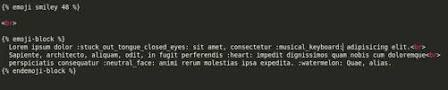# 强化学习观点下的社交推荐系统构建1. 忽略模型过程和细节
2. 样本的精细化处理
3. 过于依赖算法
4. 核心数据控制
5. 团队的全栈性
6. 系统边界模糊/巨型系统
7. 基础数据架构建设
8. 总结

# Spark-Mllib-Matrix

MLlib 中的线性代数支持有 Breeze 和 jblas 两种。

# REINFORCE 算法

## 预备知识

$\eta(\theta) = \mathbb{E}\Bigg[\sum\limits_{t=0}^{T} \gamma^t r(s_t, a_t)\Bigg]$

$\nabla_\theta \eta(\theta) = \mathbb{E}\Bigg[(\sum\limits_{t=0}^{T} \gamma^t r(s_t, a_t))(\sum\limits_{t=0}^{T}\nabla_\theta \log \pi_\theta (a_t|s_t))\Bigg]$

$\mathbb{E} [r(s_{t’},a_{t’}) \nabla_\theta \log\pi_\theta (a_t|s_t)] = 0$

$\nabla_\theta\eta(\theta) = \mathbb{E}\Bigg[\sum\limits_{t=0}^{T}\nabla_\theta\log \pi_\theta(a_t|s_t)\sum\limits_{t’=t}^{T}\gamma^{t’}r(s_{t’},a_{t’})\Bigg]$

$\nabla_\theta\eta(\theta) = \mathbb{E}\Bigg[\sum\limits_{t=0}^{T}\nabla_\theta\log \pi_\theta(a_t|s_t)\sum\limits_{t’=t}^{T}\gamma^{t’-t}r(s_{t’},a_{t’})\Bigg]$

1. 初始化参数为 $\theta_1$ 的策略 $\pi$.
2. 对迭代 $k = 1,2,\dots$:
1. 根据当前策略 $\theta_k$ 采样 $N$ 个轨迹 $\tau_1,\dots,\tau_n$，其中 $\tau_i = (s_t^i, a_t^i, R_t^i)_{t=0}^{T-1}$.注意到因为在观察到最后的状态时无行动了已经，所以最后的状态丢弃。
2. 计算经验策略梯度：$\widehat{\nabla_\theta\eta(\theta)} = \frac{1}{NT}\sum\limits_{i=1}^{N}\sum\limits_{t=0}^{T-1} \nabla_\theta\log \pi_\theta(a_t^i|s_t^i)R_t^i$
3. 进行一步梯度计算：$\theta_{k+1} = \theta_k + \alpha\widehat{\nabla_\theta\eta(\theta)}$

## 构造计算图

$\widehat{\nabla_\theta\eta(\theta)} = \nabla_\theta\Bigg(\frac{1}{NT}\sum\limits_{i=1}^{N}\sum\limits_{t=0}^{T-1}\log\pi_\theta(a_t^i|s_t^i)R_t^i \Bigg) = \nabla_\theta L(\theta)$

## 梯度更新和诊断

$\widehat{\nabla_\theta\eta(\theta)} = \frac{1}{NT}\sum\limits_{i=1}^{N}\sum\limits_{t=0}^{T-1}\nabla_\theta\log\pi_\theta(a_t^i|s_t^i)(R_t^i-b(s_t^i))$

# Inverse Reinforcement Learning for Learning the Cost Function

$$x_{t+1} = f(x_t,u_t)$$

$$V(x_1,\cdots,x_N) = \sum_{t=0}^{H} x_t^{\intercal} Q x_t + u_t^{\intercal} R u_t$$

$$Q=\begin{bmatrix} q_1 & 0 & \ldots & 0\\ 0 & q_2 & \ldots & 0\\ \vdots & \vdots & \ddots & \vdots\\ 0 & 0 &\ldots & q_n \end{bmatrix},R=\begin{bmatrix} r_1 & 0 & \ldots & 0\\ 0 & r_2 & \ldots & 0\\ \vdots & \vdots & \ddots & \vdots\\ 0 & 0 &\ldots & r_n \end{bmatrix}$$

$$x^{\intercal} Q x + u^{\intercal} R u = w^{*} \cdot \phi(x,u)~\mathrm{where}~w^{*} =\begin{bmatrix}q_1 \\ \vdots\\ q_n\\ r_1\\ \vdots\\ r_m\\ \end{bmatrix} \in \mathbb{R}^{m+n}$$

\begin{align} \mathbb{E}_{x_0 \sim I}[V^\pi(x_0)] &=\mathbb{E} \Bigg[\sum\limits_{t=0}^{H}\mathcal{R}(x_t,u_t) \Bigg]=\mathbb{E}\Bigg[\sum_{t=0}^{H} w \cdot \phi(x_t, u_t) \Bigg] \\&=w \cdot \mathbb{E}\Bigg[\sum_{t=0}^{H} \phi(x_t,u_t) \Bigg]=w \cdot \mu(\pi)\end{align}

$$\Bigg|\mathbb{E}\Bigg[\sum\limits_{t=0}^{H}\mathcal{R}(x_t,u_t)|\tilde{\pi}\Bigg]-\mathbb{E}\Bigg[\sum\limits_{t=0}^{H}\mathcal{R}(x_t,u_t)|\tilde{\pi_E}\Bigg]\Bigg| = |w^\intercal\mu(\tilde{\pi})-w^\intercal\mu_E|\leq ||w||_2||\mu(\tilde{\pi})-\mu_E||_2\leq 1\cdot \epsilon = \epsilon$$

Inverse RL 算法. 算法主要过程如下：

• 初始化：随机选择某个策略 $\pi_0$，计算（或者通过 Monte Carlo 近似）$\mu_0 = \mu(\pi_0)$
• 主要循环：
1. 对每个 $i\geq 1$，计算
$$t_i = \max_{w:||w||_2\leq 1} \min_{j\in{0,\cdots,i-1}} w^{\intercal}(\mu_E - \mu_j)$$
令 $w_i$ 为达到最大值的 $w$ 的值
2. 如果 $t_i \leq \epsilon$，终止
3. 否则，使用某个 RL 算法来计算最优策略 $\pi_i$ 使用奖励 $\mathcal{R} = w_i^{\intercal} \phi$
4. 计算（或者估计）$\mu_i = \mu(\pi_i)$
5. 设置 $i = i + 1$，回到 step 1

\begin{aligned} & \underset{t,w}{\text{minimize}} & & t \\ & \text{s.t.} & & w^{\intercal} \mu_E \geq w^{\intercal} \mu_j + t, \; j = 1, \ldots, i-1\\ & & & ||w||_2\leq 1 \end{aligned}

$$\forall w\;\mathrm{with}\;||w||_2 \leq 1\; \exists i\;\mathrm{s.t.} w^{\intercal}\mu_i \geq w^{\intercal} \mu_E - \epsilon$$

1 P. Abbeel and A. Y. Ng. Apprenticeship learning via inverse reinforcement learning. In International Conference on Machine Learning (ICML), 2004.